Addition and Subtraction of Fractions - Statement Questions

Chapter 2 Class 7 Fractions and Decimals
Concept wise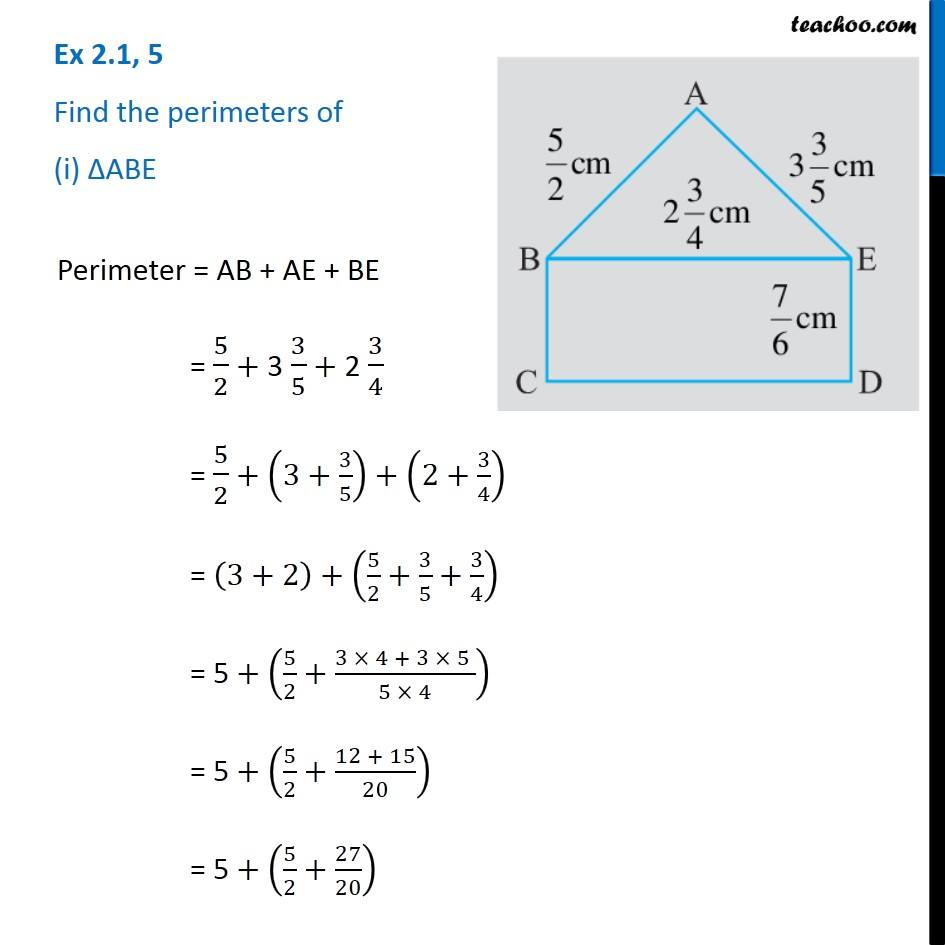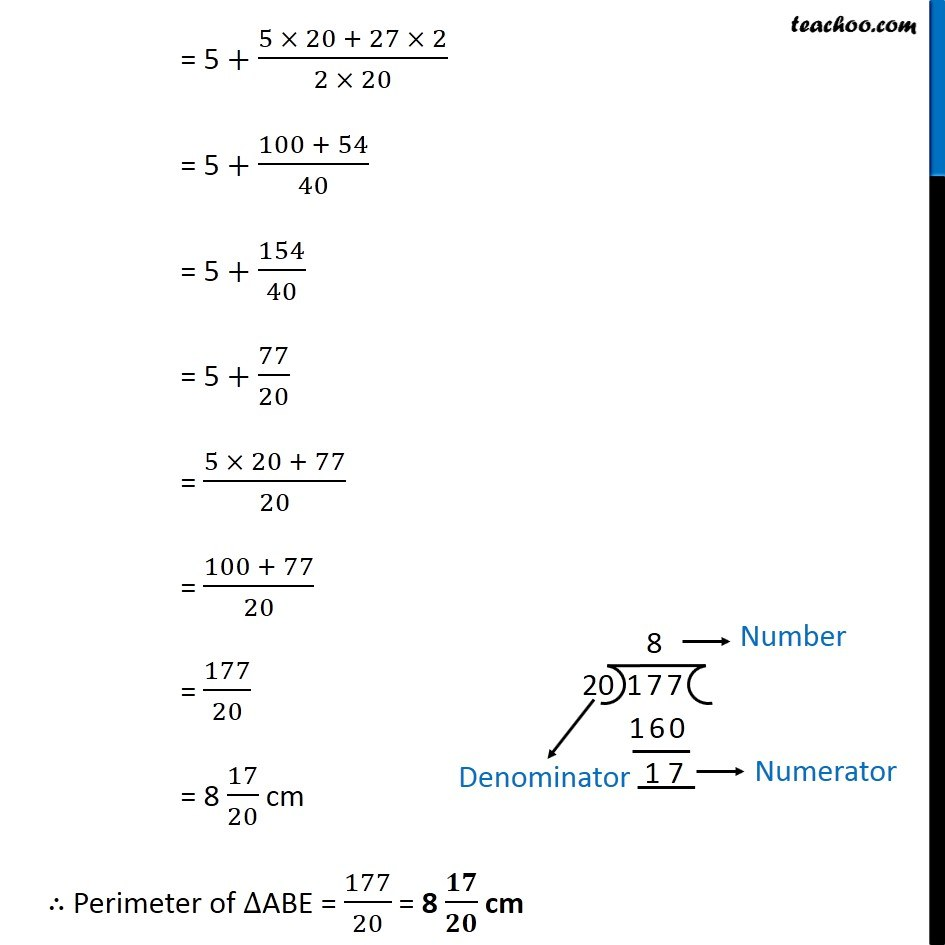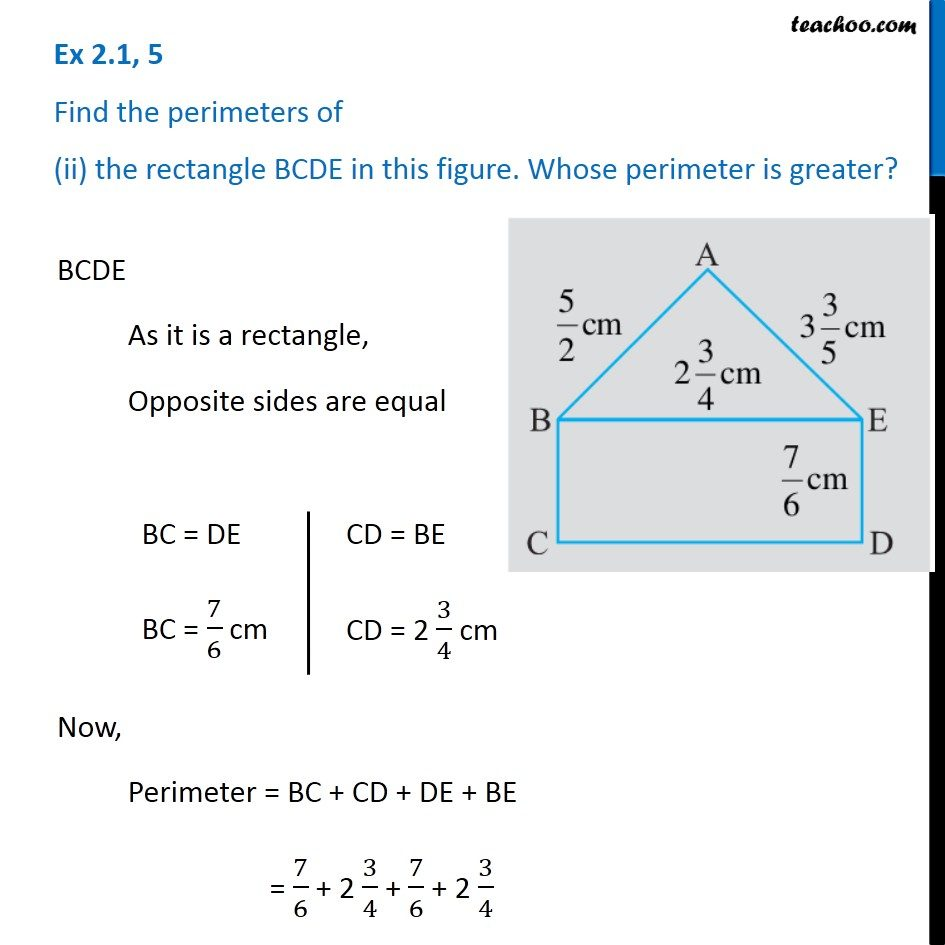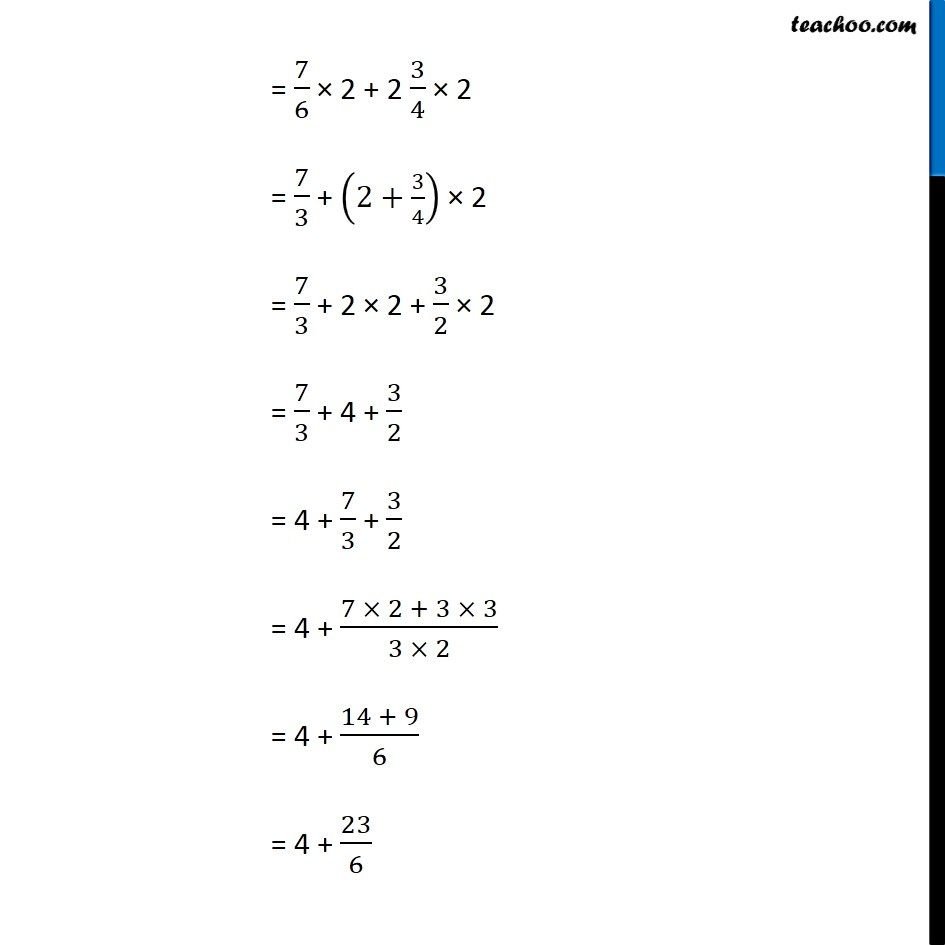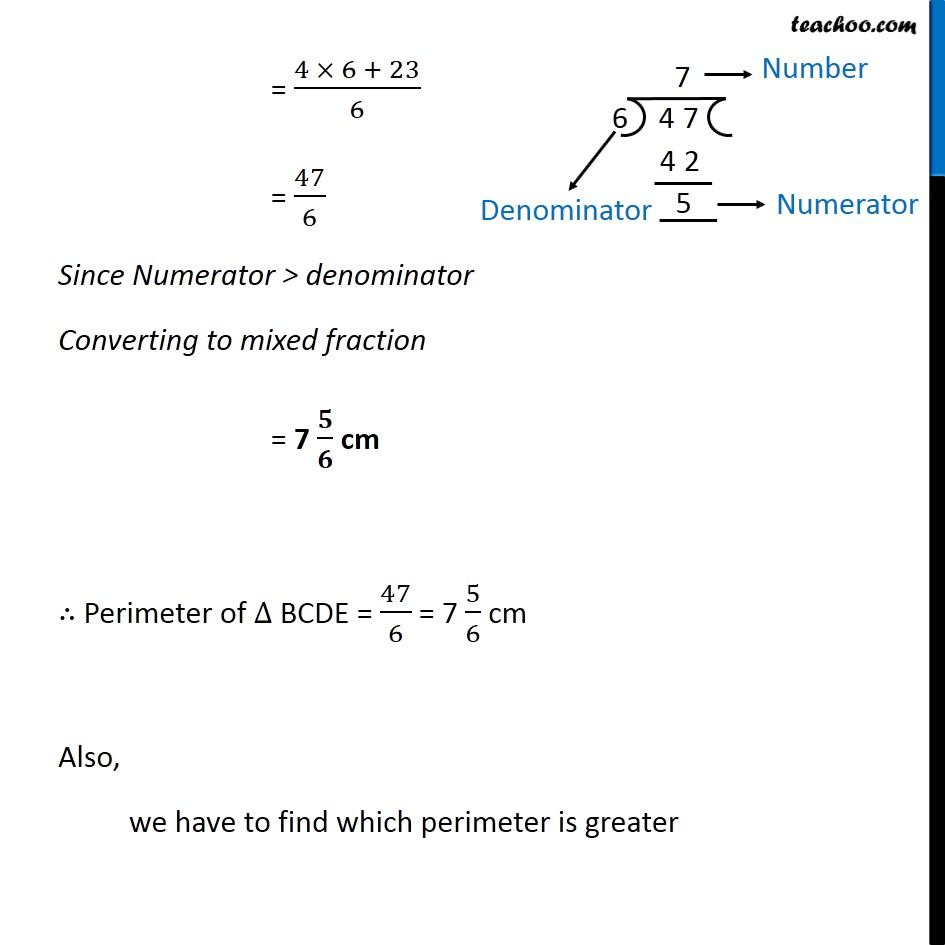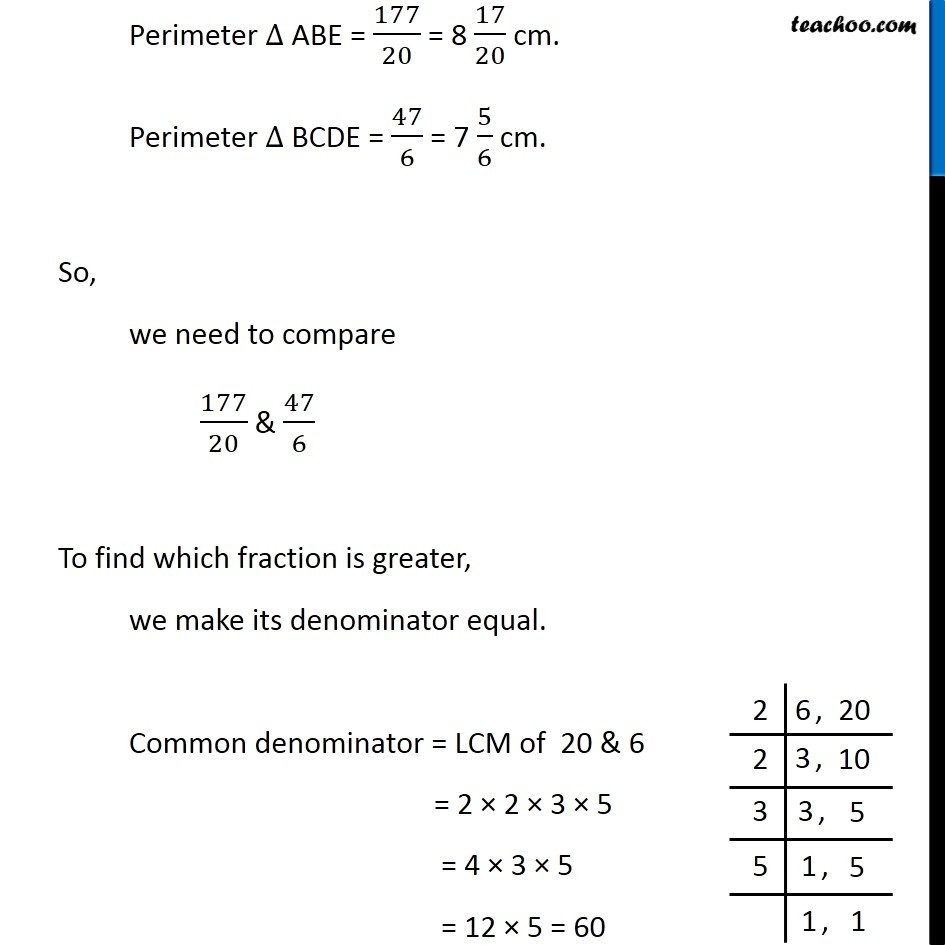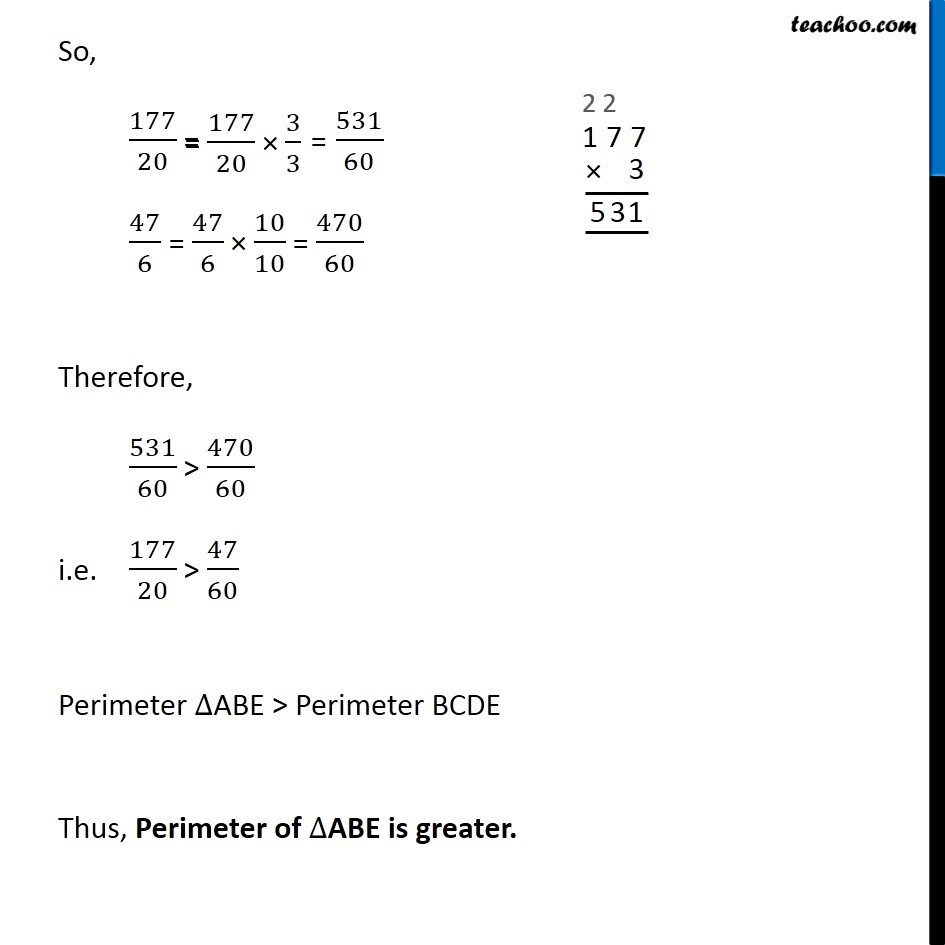### Transcript

Ex 2.1, 5 Find the perimeters of (i) ABE Perimeter = AB + AE + BE = 5/2 + 3 3/5 + 2 3/4 = 5/2 + (3+3/5)+ (2+3/4) = (3+2) + (5/2+3/5+3/4) = 5 + (5/2+(3 4 + 3 5 )/(5 4)) = 5 + (5/2+(12 + 15)/20) = 5 + (5/2+27/20) = 5 + (5 20 + 27 2)/(2 20) = 5 + (100 + 54)/40 = 5 + 154/40 = 5 + 77/20 = (5 20 + 77)/20 = (100 + 77)/20 = 177/20 = 8 17/20 cm Perimeter of ABE = 177/20 = 8 / cm Ex 2.1, 5 Find the perimeters of (ii) the rectangle BCDE in this figure. Whose perimeter is greater?BCDE As it is a rectangle, Opposite sides are equal Now, Perimeter = BC + CD + DE + BE = 7/6 + 2 3/4 + 7/6 + 2 3/4 = 7/6 2 + 2 3/4 2 = 7/3 + (2+3/4) 2 = 7/3 + 2 2 + 3/2 2 = 7/3 + 4 + 3/2 = 4 + 7/3 + 3/2 = 4 + (7 2 + 3 3)/(3 2) = 4 + (14 + 9)/6 = 4 + 23/6 = (4 6 + 23)/6 = 47/6 Since Numerator > denominator Converting to mixed fraction = 7 / cm Perimeter of BCDE = 47/6 = 7 5/6 cm Also, we have to find which perimeter is greater Perimeter ABE = 177/20 = 8 17/20 cm. Perimeter BCDE = 47/6 = 7 5/6 cm. So, we need to compare 177/20 & 47/6 To find which fraction is greater, we make its denominator equal. Common denominator = LCM of 20 & 6 = 2 2 3 5 = 4 3 5 = 12 5 = 60 So, 177/20 = 47/6 Therefore, 531/60 > 470/60 i.e. 177/20 > 47/60 Perimeter ABE > Perimeter BCDE Thus, Perimeter of ABE is greater.